# NUMBER CONVERSION USING C++ PROGRAM!!!

|DECIMAL TO BINARY||BINARY TO DECIMAL|

“Using a do-while loop creates the following menu-based c++ program.

1. Convert a Decimal to a Binary number.
2. Convert a Binary to a Decimal number.
3. Exit.

Depending on the choice accept the value and display the result. The program should continue till the user selects the third option.”

In this program, we are going to do a number conversion that means we going to convert decimal to binary and binary to decimal ;

Here we are going to do two codings in the same program using a do-while loop.

DO-WHILE LOOP:

The do-While loop is a variant of the while loop. This loop will execute the code block once, before checking if the condition is true, then it will repeat the loop as long as the condition is true.

• Let’s start the program with the header file

“#include<iostream>” →input-output continuous bytes of data.

“#include<cmath>” →this is given because we have given the function pow(power of number).

“using namespace std;” →used to store variable value.

“int main()” →returns datatype; beginning of the program execution.

• Then we going to declare the variable,

“{int dec,d,i,temp,ch;

long int bin;”

*dec →decimal number; value that we are going to get from the user.

*1 st five variable (i.e.,dec,d,i,temp,ch) are defined as integer.

*” bin” is defined as a long integer data type(accept many digits).

• Next, we are going to use the do-while loop; the do-while loop is used to repeat the execution process till we terminate.
• Now, we will initialize some variables that are equal to “0”.

“dec=bin=d=i=0;

cout<<”\n\n\t\tMENU\n1.Decimal to Binary number\n2.Binary to Decimal\n3.Exit\n”;

cin>>ch;”

In “cout” statement we have given “MENU” which means;

→if you give “1” it will execute decimal to binary.

→“2” it will execute binary to decimal.

→”3” it will exit.

→if you give any other number the cout statement will be executed again as “Enter your choice(1/2/3)”.

• Now, we are going to pass the “ch” variable into “switch(ch)”.

“{ case 1:cout<<”Enter a Decimal Number:”;

cin>>dec;

temp=dec;

while(dec!=0)

{ d=dec%2;

bin+=d*pow(10,i);

dec/=2;

i++; }

cout<<temp<<”in decimal =”<<bin<<”in binary”<<endl;break;”

• Now, in 1st case, we are going to convert the number “decimal to binary”

[For eg: take 12][bin value =1100 →you should know that!]

→Enter a decimal number:12

→So, it stores dec =12 and the same value is stored in another variable(i.e.,)temp=12;[temp is used for printing purpose]

“while(dec!=0)”

→Till the dec become “0” the calculation will be continued.

## LOGIC OF THE PROGRAM:

• “cout<<temp<<”in decimal=”<<bin<<”in binary”<<endl;break;”

It will print like 12 in decimal =1100 in binary.

*endl →avoid buffer statement and display in a new line.

*break →used to terminate the loop.

• Next, “case 2:cout<<”Enter the Binary Number:”;

cin>>bin;

temp=bin;

while(bin!=0)

{ d=bin%10;

dec+=d*pow(2,i);

bin/=10;

i++; }

cout<<temp<<”in binary =”<<dec<<”in decimal”;”

→Now in 2 nd case we are going to convert the numbers into “binary to decimal”.

NOTE: SAME AS WE HAVE DONE IN CASE 1(“DECIMAL TO BINARY”)

• Next, “case 3:break;

default:cout<<”INVALID CHOICE”;”

→in 3rd case we have defaulty given as invalid choice.

• Atlast, “while(ch!=3);

return 0;”

→This is given because, till the do while become not equal to ”3” it will continue its process ; then return 0;

--

--

--

## More from Gomathi Keerthana.R.S.

Love podcasts or audiobooks? Learn on the go with our new app.

## A note on Kentucky Mule and Twitter’s Scala compiler announcement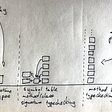## Dynamic Computation Graphs(DCG) with Tensorflow Fold!!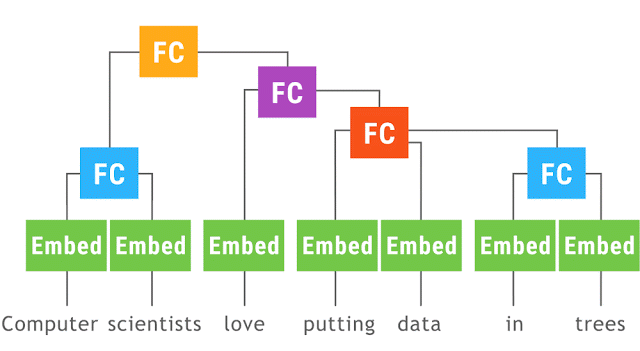## What is an API?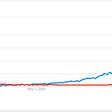## Open VNDC Farming from January 26, 2021## Skiptracing Part 2: iOS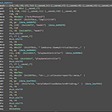## Going from coder to a builder## Building BBC Sounds in the API## App development process: A step-by-step guide## How to Fix NET::ERR_CERT_DATE_INVALID Error?## How do I manage a dependency inherited from a parent POM in the child POM : Log4j example?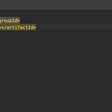## PayStub — Phishing Analysis